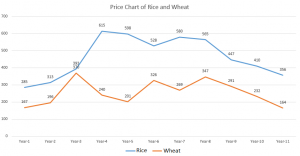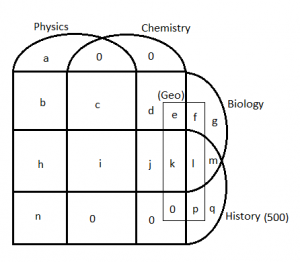# Logical Reasoning and Data Interpretation for the CAT PDF Set-2

0
16326

Logical Reasoning and Data Interpretation for the CAT PDF Set-2:

Instructions: Given in the below chart are prices(in Rupee/100kg) of rice and wheat over a period of 11 years. Answer the following questions based on the given information.Question 1: If the price of rice in year-1 is greater than that of wheat in year-1 by X% and the price of rice in year-11 is greater than that of wheat in year-11 by Y%, then by how many percentage points is X lesser than Y?

a) 13.54
b) 29.39
c) 23.1
d) None of these

Question 2: If the greatest percentage difference between the price of rice and wheat(w.r.t wheat) in any year is X and the smallest percentage difference between the price of rice and wheat(w.r.t to wheat) in any year is Y, then by what percentage(closest integer) is Y less than X?

a) 94%
b) 95%
c) 96%
d) 97%

Question 3: If M is the number of years that both the curves showed a similar trend in the prices and N is the number of years that both the curves showed different trend in the prices, then find the value of |M – N|.

a) 0
b) 2
c) 4
d) 6

Question 4: Raghu buys 100kg of rice in year 1 and sells them in year 2 at the price in year 2. He buys 100 kg of wheat in year 2 and sells them in year 3 at the price in year 3. He buys 100 kg of rice in year 3 and sells them in year 4 at the price in year 4. This process is continued until he sells 100 kg of wheat in year-11. Find the total profit percentage he gets.

a) 1.69%
b) 1.96%
c) 2.25%
d) 2.56%

Instructions: There are 1000 students in a college who study one or more subjects among History, Geography, Biology, Physics and Chemisty. 225 students study Biology but not History. 500 people study History. Exactly 325 students study three subjects and exactly 225 students study four subjects. All those students who study Geography do not study Physics. No student studies geography alone. The number of students who study all Physics, Biology and Chemisty but not the other two is half the number of students who do not study History but study Geography. Exactly 175 students study History and at most one more subject. All those students who study Chemisty also study Biology.

Question 5: How many students study only Physics?

a) 200
b) 225
c) 275
d) 300

Question 6: What is the ratio of number students who study only Physics, Chemisty and Biology to the number of students who study only Biology, Chemisty and Geography?

a) 4:1
b) 1:4
c) 2:1
d) 1:2

Question 7: If the total number of people who study Physics is 300, then what percentage of students who study Physics also study Biology and no other subject?

a) 0
b) 15
c) 20
d) 30

Question 8: What percentage of students study both Biology and Chemisty but not History?

a) 10
b) 15
c) 20
d) 22.5

X = $\frac{118}{167}*100$ = 70.6%
Y = $\frac{192}{164}*100$ = 117%
Percentage points difference => Y – X = 117 – 70.6 = 46.4

We can see that the greatest price difference is in year 5 => Percentage difference = $\frac{598-201}{201}*100$ = 197.5%
Least percentage difference is in year-3 => percentage difference = $\frac{23}{370}*100$ = 6.2%
The difference = 197.5 – 6.2 = 191.3
% difference = $\frac{191.3}{197.5}*100$ = 97%

We can see that the prices of both the commodities either increase or decrease in 6 years
=> M = 6
The price of one of the commodity increases and the price of the other decreases in 4 years
=> N = 4
Hence, |M – N| = 2

Total cost price of Rice = 285 + 393 + 598 + 580 + 447 = Rs 2303
Total selling price of Rice = 313 + 615 + 528 + 565 + 410 = Rs 2431
Total cost price of Wheat = 196 + 240 + 326 + 347 + 232 = Rs 1341
Total selling price of Wheat = 370 + 201 + 269 + 291 + 164 = Rs 1295
Total cost price = 2303 + 1341 = 3644
Total selling price = 2431 + 1295 = Rs 3726
Total Profit = 3726 – 3644 = Rs 82
Profit % = $\frac{82}{3644}*100$ = 2.25%

We first create a grid for intersection of 4 sets. We know that all those who study Geography also study another subject and none of them studies Physics. Hence, we can add a rectangle as shown below to the grid. We know that all those who study Chemistry also study Biology. Hence, we can put a 0 in all the 5 places which have Chemistry but no Biology. The remaining regions have been marked from a – q (no O). Hence, we get the venn diagram as follows:c + e + h + j + l = 325
b + c + d + e + f + g = 225
k + i = 225
m + n + p + q = 175
2c = e + f
We need to find a.
a = 1000 – (Students who study History) – (b + c + d + e + f + g)
=> a = 1000 – 500 – 225 = 275

c + e + h + j + l = 325
b + c + d + e + f + g = 225
k + i = 225
m + n + p + q = 175
2c = e + f
We need to find c:e.
h + i + j + k + l = 200 – (m + n + p + q)
=> h + i + j + k + l = 500 – 175 = 325
=> h + j + l = 325 – 225 = 100
=> c + e = 325 – 100 = 225
=> b + d + f + g = 225 – 225 = 0
=> b = d = f = g = 0
=> 2c = e
=> c : e = 1 : 2

c + e + h + j + l = 325
b + c + d + e + f + g = 225
k + i = 225
m + n + p + q = 175
2c = e + f
We need to find c:e.
h + i + j + k + l = 200 – (m + n + p + q)
=> h + i + j + k + l = 500 – 175 = 325
=> h + j + l = 325 – 225 = 100
=> c + e = 325 – 100 = 225
=> b + d + f + g = 225 – 225 = 0
=> b = d = f = g = 0
As b is 0, 0% of people study only Physics and Biology.

c + e + h + j + l = 325
b + c + d + e + f + g = 225
k + i = 225
m + n + p + q = 175
2c = e + f
We need to find c:e.
h + i + j + k + l = 200 – (m + n + p + q)
=> h + i + j + k + l = 500 – 175 = 325
=> h + j + l = 325 – 225 = 100
=> c + e = 325 – 100 = 225
=> b + d + f + g = 225 – 225 = 0
=> b = d = f = g = 0
=> 2c = e
=> c : e = 1 : 2
=> c = 75 and e = 150
students who study both Biology and Chemisty but not History = c + d + e + f + g = 75 + 0 + 150 + 0 + 0 = 225